Chemical-Kinetics-Previous-Year

# Important Questions of Chemical Kinetics for NEET Chemistry

1. For the chemical reaction,  N2(g) + 3H2(g) 2NH3(g) the correct option is  (NEET 2019)

(a)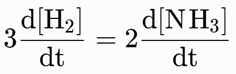(b)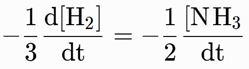(c)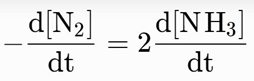(d)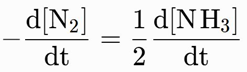2.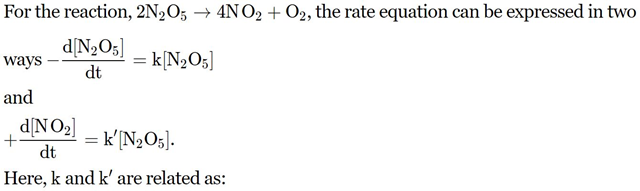(Mains 2011)

(a) k’ = 2k, k’’=  k         (b)k’ = 2k, k’’ = k / 2

(c) k’ = 2k, k’’ = 2k        (d) k’ = k, k’’ = k

3. For the reaction N2O5(g) 2NO2(g) + ½ O2(g) the value of rate of disappearance of N2O5 is given as 6.25 × 10–3 mol L–1s–1. The rate of formation of NO2 and O2 is given respectively as :    (2010)

(a) 6.25 × 10–3 mol L–1 s–1 and 6.25 × 10–3 mol L–1 s–1

(b) 1.25 × 10–2 mol L–1 s–1 and 3.125 × 10–3 mol L–1 s–1

(c) 6.25 × 10–3 mol L–1 s–1 and 3.125 × 10–3 mol L–1 s–1

(d) 1.25 × 10–2 mol L–1 s–1 and 6.25 × 10–3 mol L–1 s–1

4.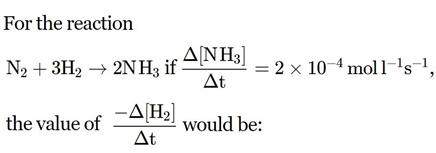(2009)

(a) 4 × 10–4 mol L–1 s–1            (b) 6 × 10–4 mol L–1 s–1

(c) 1 × 10–4 mol L–1 s–1            (d) 3 × 10–4 mol L–1 s–1

5.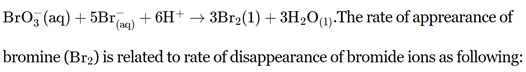(2009)

(a)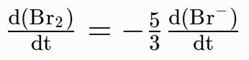(b)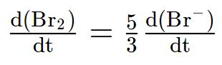(c)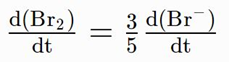(d)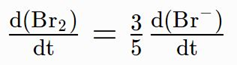6.